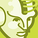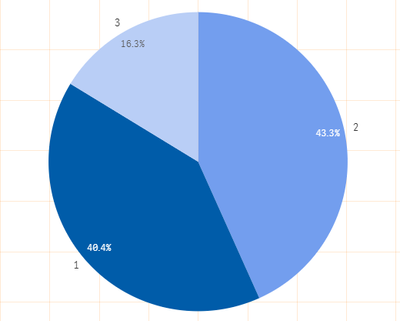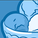# App Development

Announcements
cancel
Showing results for
Did you mean:Contributor III

## Change Dimension labels in pide chart

Hello,

I have a pie chart to represent 3 percentages. The label on each of the pie portions are showing the level number (1,2 or 3), and I want to show the total value of each portion of the chart.

Here is the chart:I want to change the labels 1,2 and 3 by fro example, 1200 in place of 1, 1290 in place of 2 and 510 in place of 3.

I have tried to write a set expression in the box "label" of the Dimension. but nothing changed.

Thank you!

Labels (1)
• ### expression

1 Solution

Accepted SolutionsSpecialist

In the dimension expression (not the label), use this:

=aggr(Count({1<DQ_TYPE={1,2,3,4},EXEC_DATE_SQL={">\$(fecha_estudio)"},ACCOUNT_TYPE_ID={'C'}>} ID_DQ_FACTS), [Level fieldname])

You could also include the Level value and the count like this:

=aggr([Level fieldname]&' - '&Count({1<DQ_TYPE={1,2,3,4},EXEC_DATE_SQL={">\$(fecha_estudio)"},ACCOUNT_TYPE_ID={'C'}>} ID_DQ_FACTS), [Level fieldname])

3 RepliesSpecialist

Since the level is a sequential number starting with 1, you can use the pick() function, like this:

=Pick([Level Number],'1200','1290','510')

You may need to replace [Level Number] with appropriate field name.

If [Level Number] was not a sequential number, you would use a combination of pick() and match(), like this:

=Pick(Match([Level],'value 1','value 2','value 3'),'1200','1290','510')

Use this as your dimension expression.Contributor III
Author

Thank you Gary,

it is not a secuential number, it should be the total of the portion of the pie chart. The numbers were examples.

If, for example, we have a total of 3000, the 40% is 1290, so i want to show the 40% inside the pie chart and the 1290 in the label. It would also be OK to show the totals in a legend, but i don't know how to do that.

For more information, the formula that i tried to use in the "label" of the Dimensions of the pie chart is this:

Count({1<DQ_TYPE={1,2,3,4},EXEC_DATE_SQL={">\$(fecha_estudio)"},ACCOUNT_TYPE_ID={'C'}>} ID_DQ_FACTS)

But that did nothing.

Thank you again.Specialist

In the dimension expression (not the label), use this:

=aggr(Count({1<DQ_TYPE={1,2,3,4},EXEC_DATE_SQL={">\$(fecha_estudio)"},ACCOUNT_TYPE_ID={'C'}>} ID_DQ_FACTS), [Level fieldname])

You could also include the Level value and the count like this:

=aggr([Level fieldname]&' - '&Count({1<DQ_TYPE={1,2,3,4},EXEC_DATE_SQL={">\$(fecha_estudio)"},ACCOUNT_TYPE_ID={'C'}>} ID_DQ_FACTS), [Level fieldname])